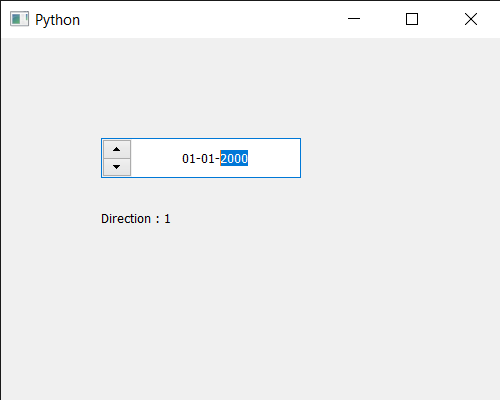Related Articles

# PyQt5 QDateEdit – Getting Direction

• Last Updated : 07 Jul, 2020

In this article we will see how we can get the direction of the date edit. By default when we create a date edit arrow buttons are at the right side although we can change it, by changing the layout direction with the help of `setLayoutDirection` method.

In order to do this we use `layoutDirection` method with the QDateEdit object

Syntax : date.layoutDirection()

Argument : It takes no argument

Return : It returns Direction object but when printed it shows the associated value with it

Below is the implementation

 `# importing libraries``from` `PyQt5.QtWidgets ``import` `*` `from` `PyQt5 ``import` `QtCore, QtGui``from` `PyQt5.QtGui ``import` `*` `from` `PyQt5.QtCore ``import` `*` `import` `sys`` ` ` ` `class` `Window(QMainWindow):`` ` `    ``def` `__init__(``self``):``        ``super``().__init__()`` ` `        ``# setting title``        ``self``.setWindowTitle(``"Python "``)`` ` `        ``# setting geometry``        ``self``.setGeometry(``100``, ``100``, ``500``, ``400``)`` ` `        ``# calling method``        ``self``.UiComponents()`` ` `        ``# showing all the widgets``        ``self``.show()`` ` `    ``# method for components``    ``def` `UiComponents(``self``):`` ` `        ``# creating a QDateEdit widget``        ``date ``=` `QDateEdit(``self``)`` ` `        ``# setting geometry of the date edit``        ``date.setGeometry(``100``, ``100``, ``200``, ``40``)`` ` `        ``# alignment``        ``a_flag ``=` `Qt.AlignCenter`` ` `        ``# setting alignment of date``        ``date.setAlignment(a_flag)``         ` `        ``# setting layout direction``        ``date.setLayoutDirection(Qt.RightToLeft)`` ` ` ` `        ``# creating a label``        ``label ``=` `QLabel(``"GeeksforGeeks"``, ``self``)`` ` `        ``# setting geometry``        ``label.setGeometry(``100``, ``150``, ``250``, ``60``)`` ` `        ``# making label multiline``        ``label.setWordWrap(``True``)`` ` `        ``# getting direction``        ``value ``=` `date.layoutDirection()`` ` ` ` `        ``# setting text to the label``        ``label.setText(``"Direction : "` `+` `str``(value))`` ` ` ` `# create pyqt5 app``App ``=` `QApplication(sys.argv)`` ` `# create the instance of our Window``window ``=` `Window()`` ` `# start the app``sys.exit(App.``exec``())`

Output :Attention geek! Strengthen your foundations with the Python Programming Foundation Course and learn the basics.

To begin with, your interview preparations Enhance your Data Structures concepts with the Python DS Course. And to begin with your Machine Learning Journey, join the Machine Learning – Basic Level Course

My Personal Notes arrow_drop_up